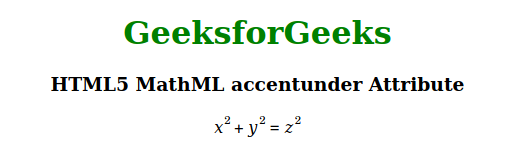# HTML5 MathML accentunder Attribute

• Last Updated : 18 Nov, 2020

The MathML accentunder attribute is used to specify whether an element is treated as an accent or not. A value of true means that the accent is drawn closer to the base expression and a value of false means that a limit is drawn instead. The difference between the two is that a limit is reduced in size whereas an accent is the same size as the base.

Syntax:

`<element accentunder="true | false">`

Attributes Values: This attribute has two values as mentioned above and described below:

• true: This value means that the element is treated as an accent that is drawn closer to the base expression.
• false: This value means that the element is treated as a limit.

The example below illustrates the MathML accentunder attribute:

Example:

## HTML

 ```<``html``>`` ` `<``body` `style``=``"text-align:center;"``>`` ` `    ``<``h1` `style``=``"color:green"``>``        ``GeeksforGeeks``    ```` ` `    ``<``h3``>``        ``HTML5 MathML accentunder Attribute``    ```` ` `    ``<``math``>``        ``<``mrow``>``            ``<``mrow``>``                ``<``msup``>``                    ``<``mi``>x``                    ``<``mn``>2``                ````                ``<``mo` `accentunder``=``"true"``>+``                ``<``msup``>``                    ``<``mi``>y``                    ``<``mn``>2``                ````            ````            ``<``mo` `accentunder``=``"false"``>=``            ``<``msup``>``                ``<``mi``>z``                ``<``mn``>2``            ````        ````    `````` ` ``

Output:Supported Browsers: The browsers supported by HTML5 MathML accentunder attribute are listed below:

• Firefox

My Personal Notes arrow_drop_up